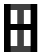## The Alphabet Game

The alphabet game is a game I learned as a preteen in New York City from a now-forgotten substitute teacher. This is how you play:
Each letter in the alphabet is assigned a number (a=1, b=2, c=3, and so on);
The player's task is to find a word whose letters add up to exactly 100.

For example: the word "apple" has a score of 50.
a (1) + p (16) + p (16) + l (12) + e (5) = 50